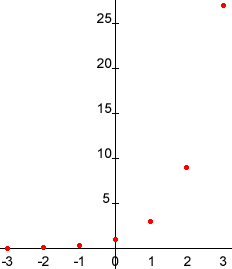jose grade 11 student

graph the exponential problem F(x)=3x

Hi Jose,

Set up a table of values as you would for graphing other functions. For example

xF(x)
131 = 3
232 = 9
333 = 27

For negative values of x can use the fact that a-b = 1/ab and extend the table to

xF(x)
131 = 3
232 = 9
333 = 27
-13-1 = 1/3
-23-2 = 1/9
-33-3 = 1/27

Also 30 = 1 so the final version of my table is

xF(x)
131 = 3
232 = 9
333 = 27
-13-1 = 1/3
-23-2 = 1/9
-33-3 = 1/27
030 = 1

Now plot the points in the coordinate plane.Finally draw a smooth curve joining the points.

Cheers,
Harley
Go to Math Central